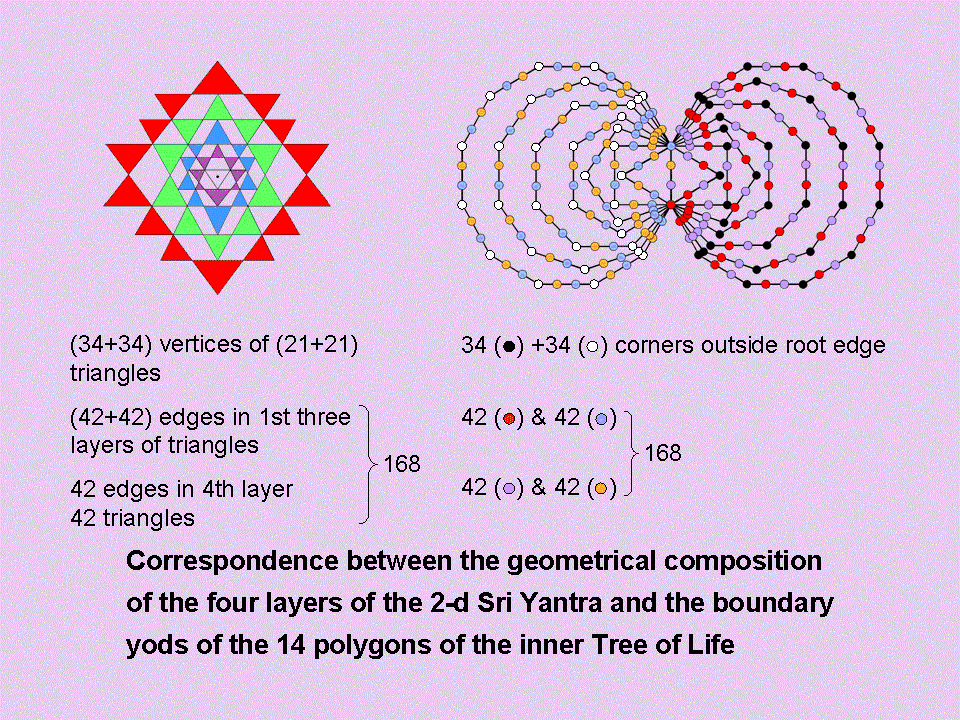Home

 << Previous    1...   7  8    10  11  ...39    Next >>#9 Parallelism between the Inner Tree of Life and the 2-d Sri Yantra

This displays in more detail the correspondence between the 236 geometrical elements in the 42 triangles of the 2-dimensional Sri Yantra and the 236 yods that line the sides of the 14 regular polygons in the inner Tree of Life. The counterpart of the two halves of the former is the two halves of the latter, although it must be noted that the 21 triangles in one half of the Sri Yantra are not precisely the mirror image of the 21 triangles in its opposite half, as is the case for the two halves of the inner Tree of Life. Corners of triangles correspond to corners of polygons and sides in the first three sets of triangles correspond to red & blue hexagonal yods. The purple yods do not denote solely either triangles or sides in the fourth set of triangles because this would destroy the 1:1 correspondence between the two halves of each system. Instead, the 42 purple yods denote the 21 triangles and the 21 sides in one half of the fourth set of triangles, whilst the 42 orange yods denote the 21 triangles and 21 sides in the fourth set of triangles in the other half. Notice that — as required by the analogy with the Sri Yantra — the 42 yods of each colour divide up into two sets of 21 yods. One set consists of one yod on the root edge and 20 yods that belong to the decagon & dodecagon, and the other set comprises yods that belong to the triangle, square, pentagon, hexagon & octagon. Such exact parallelism between the two systems discredits the possibility of coincidence as implausible.

 << Previous    1...   7  8    10  11  ...39    Next >>

Home